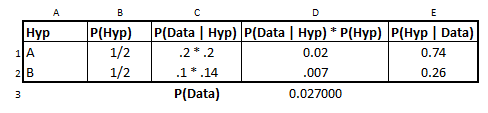# Basics, Thinking in Tables

## Review

If your Stats 425 is still kicking around in your head, you might remember Bayes’ Theorem. Which generalizes a nice, symmetric property of Conditional Probability

$P(A|B)P(B) = P(B|A) P(A)$

Into the following

$p(H|D) = \frac{p(H)~p(D|H)}{p(D)}$

Where H is your Hypothesis and D is your Data.

### Bowls

In a trivial example, we’ve got two identical bags with colored stones inside.

Bag 1 has 10 white stones and 20 black stones.
Bag 2 has 15 white stones and 15 black stones.

If we picked a bag at random and pulled out a white stone, what's the probability that we selected from Bag 1?


The trick to these problems is to swap the values from the story problem into the Bayes’ equation:

• Our Hypothesis is “Selected from Bag 1”
• Our Data is “Drew a white stone”

$p(B1|W) = \frac{p(B1)~p(W|B1)}{p(W)}$

We’ll assume either bag is as likely and use the proportion of Bag 1’s contents

$p(B1|W) = \frac{(12)~(13)}{p(W)}$

The only trick is figuring out the value of P(W), which can be expressed by blowing out the exhaustive conditional probabilities in the denominator

$p(B1|W) = \frac{(12)~(13)}{P(W|B1)P(B1) + P(W|B2)P(B2)}$

$p(B1|W) = \frac{(12)~(13)}{(13)(12) + (12)(12)}$

Solving, we get

$p(B1|W) = 0.4$

## Tables

As your hypothesis gets more complicated, however, Allen Downey’s book Think Bayes introduces structuring the problem via a helpful table to assist with the bookkeeping.

### M&M

The problem that introduces these tables looks like the following:

We’ve got two bags of M&Ms from two different years, where the color distribution found in each bag was

Bag 1994 1996
Blue .24
Brown .3 .13
Green .1 .2
Orange .1 .16
Tan .1
Red .2 .13
Yellow .2 .14

Then, you take an M&M from each bag, and first one is yellow, the second is green.

What is the probability that the yellow M&M came from the 1994 bag?

Equivalently, this also means that the green M&M also came from the 1996 bag. In lieu of writing out the fraction as above, then deconstructing the denominator into all exhaustive probabilities for selecting yellow from either bag and green from either bag, we’ll use the “Table Method.”

But first, we’ll restate the problem in terms of hypotheses.

• A: Bag 1 is from 1994, Bag 2 is from 1996
• B: Bag 1 is from 1996, Bag 2 is from 1994

We take the yellow, green pick as given and construct our table.

from IPython.display import Image

Image('images/mnm.PNG')• Each bag is equally likely, which explains column B
• The values in each cell for column C are the probability of each draw, given that hypothesis. e.g. Assuming Bag 1 is 1994, we had a .2 chance to draw yellow and a .2 chance to draw green from Bag 2, assumed to be from 1996.
• Column D is just plug and chug
• The value of D3 is the column sum, or that exhaustive probability we didn’t want to do by hand above
• Finally, column E is just dividing D through by the column total, which completes the form of the Bayesian Equation and normalizes the data.